Note: This is an archvied version of our old webpage. Some links might be broken. The current one can be found here.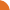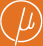Chair for Foundations of Software Reliability and Theoretical Computer Science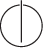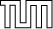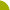Publications - Convergence Thresholds of Newton's Method for Monotone Polynomial EquationsReference:

Javier Esparza, Stefan Kiefer, and Michael Luttenberger. Convergence thresholds of Newton's method for monotone polynomial equations. In Pascal Weil and Susanne Albers, editors, Proceedings of the 25th International Symposium on Theoretical Aspects of Computer Science (STACS), pages 289–300, Bordeaux, France, 2008. Available at http://arxiv.org/abs/0802.2856.

Abstract:

Monotone systems of polynomial equations (MSPEs) are systems of fixed-point equations X_1 = f_1(X_1, ..., X_n), ..., X_n = f_n(X_1, ..., X_n) where each f_i is a polynomial with positive real coefficients. The question of computing the least non-negative solution of a given MSPE X = f(X) arises naturally in the analysis of stochastic models like stochastic context-free grammars, probabilistic pushdown automata, and back-button processes. Etessami and Yannakakis have recently adapted Newton's iterative method to MSPEs. In a previous paper we have proved the existence for strongly connected MSPEs (scMSPEs) of a threshold k_f such that after k_f iterations of Newton's method each new iteration computes at least 1 new bit of the solution. However, the proof was purely existential. In this paper we give an upper bound for k_f as a function of the maximal and minimal components of f. Using this result we show that k_f is at most single exponential resp. linear for scMSPEs derived from probabilistic pushdown automata resp. from back-button processes. Further, we prove the existence of a threshold for arbitrary MSPEs after which each new iteration computes at least 1/(w 2^h) new bits of the solution, where w, h are the width and height of the DAG of strongly connected components.

Suggested BibTeX entry:

@inproceedings{EKL08:stacs,GZipped PostScript (210 kB)PDF (199 kB)See arxiv.org ...Tech report version